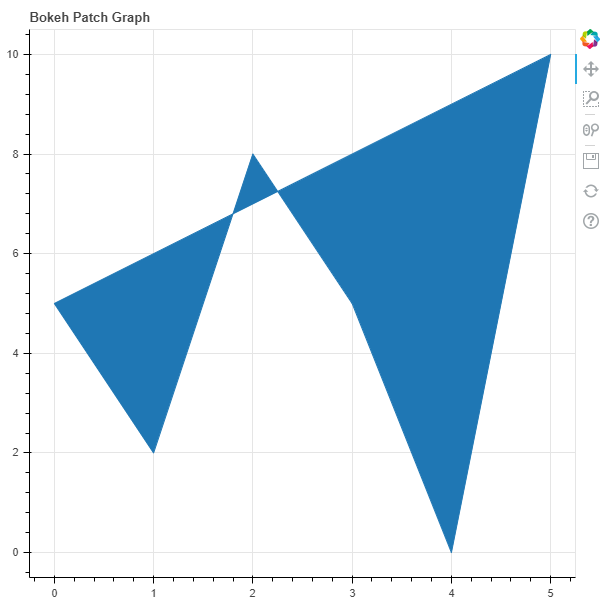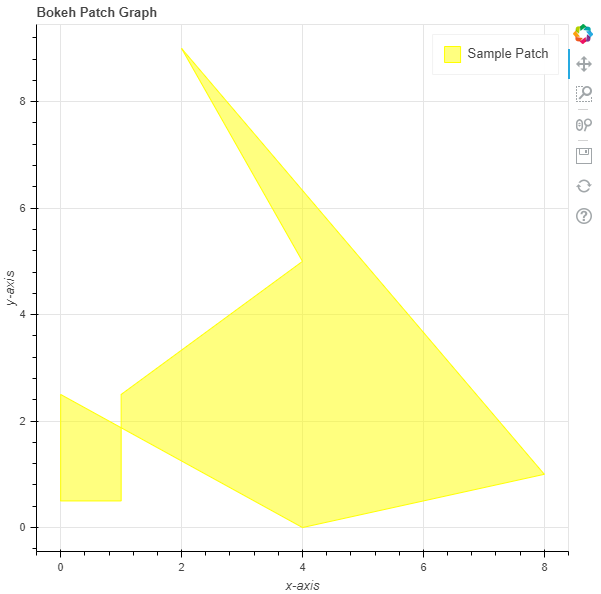GFG App
Open AppBrowser
Continue

# Python Bokeh – Plotting Patches on a Graph

Bokeh is a Python interactive data visualization. It renders its plots using HTML and JavaScript. It targets modern web browsers for presentation providing elegant, concise construction of novel graphics with high-performance interactivity.

Bokeh can be used to plot patches on a graph. Plotting patches on a graph can be done using the `patch()` method of the `plotting` module.

## plotting.figure.patch()

Syntax : patch(parameters)

Parameters :

• x : x-coordinates of the patch
• y : y-coordinates of the patch

Returns : an object of class `GlyphRenderer`

Example 1 : In this example we will be using the default values for plotting the graph.

 `# importing the modules  ` `from` `bokeh.plotting ``import` `figure, output_file, show  ` ` `  `# file to save the model  ` `output_file(``"gfg.html"``)  ` `      `  `# instantiating the figure object  ` `graph ``=` `figure(title ``=` `"Bokeh Patch Graph"``)  ` `    `  `# the points to be plotted ` `x ``=` `[``0``, ``1``, ``2``, ``3``, ``4``, ``5``] ` `y ``=` `[``5``, ``2``, ``8``, ``5``, ``0``, ``10``] ` `     `  `# plotting the graph  ` `graph.patch(x, y)  ` `      `  `# displaying the model  ` `show(graph) `

Output :Example 2 : In this example we will be plotting the patch with various other parameters

 `# importing the modules  ` `from` `bokeh.plotting ``import` `figure, output_file, show  ` ` `  `# file to save the model  ` `output_file(``"gfg.html"``)  ` `      `  `# instantiating the figure object  ` `graph ``=` `figure(title ``=` `"Bokeh Patch Graph"``)  ` ` `  `# name of the x-axis  ` `graph.xaxis.axis_label ``=` `"x-axis"` `      `  `# name of the y-axis  ` `graph.yaxis.axis_label ``=` `"y-axis"` ` `  `# points to be plotted ` `x ``=` `[``0``, ``0``, ``1``, ``1``, ``4``, ``2``, ``8``, ``4``] ` `y ``=` `[``2.5``, ``0.5``, ``0.5``, ``2.5``, ``5``, ``9``, ``1``, ``0``] ` ` `  `# color value of the patch ` `color ``=` `"yellow"` ` `  `# fill alpha value of the patch ` `fill_alpha ``=` `0.5` ` `  `# name of the legend ` `legend_label ``=` `"Sample Patch"` ` `  `# plotting the graph  ` `graph.patch(x, y, ` `            ``color ``=` `color, ` `            ``fill_alpha ``=` `fill_alpha, ` `            ``legend_label ``=` `legend_label)  ` `      `  `# displaying the model  ` `show(graph) `

Output :My Personal Notes arrow_drop_up